# Clocks

How long track travel the second hand in 46 hours, the end of which is 4 cm long.

Correct result:

x =  693.66 m

#### Solution: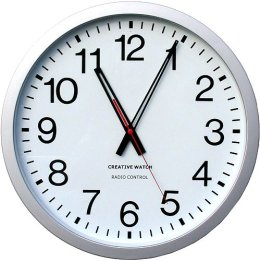We would be pleased if you find an error in the word problem, spelling mistakes, or inaccuracies and send it to us. Thank you!Tips to related online calculators
Do you want to convert length units?
Do you want to convert time units like minutes to seconds?

#### You need to know the following knowledge to solve this word math problem:

We encourage you to watch this tutorial video on this math problem:

## Next similar math problems:

• ClocksHow long path will walk the end of the clock's minute-hand long 7 cm for 330 minutes? How long will walk in the same time hour-hand?
• Clock handsThe second hand has a length of 1.5 cm. How long does the endpoint of this hand travel in one day?
• Minute-hand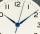How long distance will travel a large (minute) hand on the clock for 48 minutes, if its length is 56 cm?
• Clock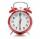What distance will pass end of 8 cm long hour hand for 15 minutes?
• Clock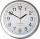How long is trajectory of second hand of hours for day, if is 15 mm long?
• Clocks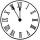What distance will describe the tip of minute hand 6 cm long for 20 minutes when we know the starting position with finally enclose hands each other 120°?
• Central angleWhat is the length of the arc of a circle with a diameter of 46 cm, which belongs to a central angle of 30°?
• Athlete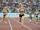How long length run athlete when the track is circular shape of radius 120 meters and an athlete runs five times in the circuit?
• Diameter of a cylinderThe diameter of the cylinder is 42 cm. How many times does the cylinder turn on a 66m long track?
• HandsThe clock shows 12 hours. After how many minutes will angle between the hour and minute hand 90°? Consider the continuous movement of both hands hours.
• Cyclist 9A cyclist travels at a a speed of 4.25 km per hour. At that rate, how far can he travel in 3.75 hours?What is the diameter of a tree around which is 3m 9dm 7cm long rope wrapped? How big is its cross-sectional area?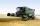Harvester has cutting width 4 m and harvested grain in the field, which is 87 meters wide. Harvester moves along the length of the field for six minutes, going back (without cutting) it takes half the time. Each turn at the end field take one minute. How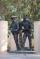There are two diggers. One digger digs a pit 46 hours second gidding 2 times faster. a) how long took to dig a pit second digger b) how long took dig together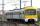On double track line between stations K and M went against each other two trains. The first train passed the distance between stations for 3.5 hours, the second, which had an average speed of 12 km/h more passed for 3.05 hours. Calculate the distance betwThe train slowed down from 90 km/h to 72 km/h in 5 seconds. How long track travel?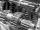In the workshop are three machines. The first is treated 250 parts for 4.7 hours, the second is treated 300 parts for 1.9 hours and the third is treated 230 parts for 4.6 hours. How long will take to make 3100 parts if worked all three machines at the sam